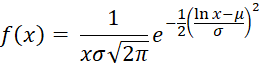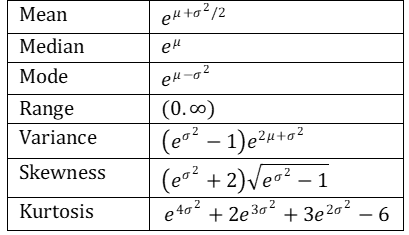# Log-normal Distribution

Definition 1: A random variable x is log-normally distributed provided the natural log of x, ln x, is normally distributed. The probability density function (pdf) of the log-normal distribution isObservation: Some key statistical properties are:Observation: Sometimes it is useful to use a transformation of the population being studied. In particular, since the normal distribution has very desirable properties, transforming a random variable into a variable that is normally distributed by taking the natural log can be useful.

Figure 1 shows a chart of the log-normal distribution with mean 0 and standard deviations 1, .5 and .25.Figure 1 – Chart of Log-normal Distribution

Note that the log-normal distribution is not symmetric, but is skewed to the right. If you have data that is skewed to the right that fits the log-normal distribution, you may be able to access various tests described elsewhere in this website that require data to be normally distributed.

Excel Functions: Excel provides the following two functions:

LOGNORM.DIST(xμσ, cum) = the log-normal cumulative distribution function with mean μ and standard deviation σ at x if cum = TRUE and the probability density function of the log-normal distribution if cum = FALSE.

LOGNORM.INV(p, μ, σ) = the inverse of LOGNORM.DIST(x, μσ, TRUE)

Note that:

LOGNORM.DIST(xμ, σ, TRUE) = NORM.DIST(LN(x), μ, σ, TRUE)

LOGNORM.DIST(xμ, σ, FALSE) = NORM.DIST(LN(x), μ, σ, FALSE)/x

LOGNORM.INV(pμ, σ) = EXP(NORM.INV(pμ, σ))

These functions are not available in versions of Excel prior to Excel 2010. Instead, these versions of Excel use LOGNORMDIST(xμ, σ), which is equivalent to LOGNORM.DIST(xμ, σ, TRUE), and LOGINV(p, μ, σ), which is equivalent to LOGNORM.INV(p, μ, σ).  For the pdf function, the formula equivalent to LOGNORM.DIST(xμ, σ, FALSE) is NORMDIST(LN(x), μ, σ, FALSE)/x.

Applied Data Science Coding: How to get class distribution in Data

# Personal Career & Learning Guide for Data Analyst, Data Engineer and Data Scientist

## Applied Machine Learning & Data Science Projects and Coding Recipes for Beginners

A list of FREE programming examples together with eTutorials & eBooks @ SETScholars

# Projects and Coding Recipes, eTutorials and eBooks: The best All-in-One resources for Data Analyst, Data Scientist, Machine Learning Engineer and Software Developer

Topics included: Classification, Clustering, Regression, Forecasting, Algorithms, Data Structures, Data Analytics & Data Science, Deep Learning, Machine Learning, Programming Languages and Software Tools & Packages.
(Discount is valid for limited time only)`Disclaimer: The information and code presented within this recipe/tutorial is only for educational and coaching purposes for beginners and developers. Anyone can practice and apply the recipe/tutorial presented here, but the reader is taking full responsibility for his/her actions. The author (content curator) of this recipe (code / program) has made every effort to ensure the accuracy of the information was correct at time of publication. The author (content curator) does not assume and hereby disclaims any liability to any party for any loss, damage, or disruption caused by errors or omissions, whether such errors or omissions result from accident, negligence, or any other cause. The information presented here could also be found in public knowledge domains.`

# Learn by Coding: v-Tutorials on Applied Machine Learning and Data Science for Beginners

Please do not waste your valuable time by watching videos, rather use end-to-end (Python and R) recipes from Professional Data Scientists to practice coding, and land the most demandable jobs in the fields of Predictive analytics & AI (Machine Learning and Data Science).

The objective is to guide the developers & analysts to “Learn how to Code” for Applied AI using end-to-end coding solutions, and unlock the world of opportunities!